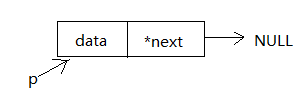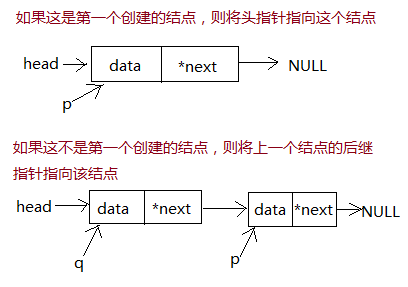# C语言创建链表

链表的结点左边一部分是存放的数据，右边一部分是后继指针指向下一个结点的地址。C语言中通常定义一个结构体类型来存储一个结点，如下：

struct node
{
int data;
struce node *next; //下一个结点的类型也是struct node，所以后继指针的类型也必须是struct node *
};

struct node *head;
head=NULL;  //头指针初始为空

现在我们来创建第一个结点，并用临时指针p指向这个结点。struct node *p;
p=(struct node *)malloc(sizeof(struct node));  //为新结点动态申请一个空间，并用临时结点p指向这个新地址
scanf("%d",&a);  //读取数据
p->data=a; //将数据存储到当前结点的data域中
p->next=NULL;  //设置当前结点的后继指针指向空，也就是当前结点的下一个结点为空

把新加入的结点串进链表。如果该结点是创建的第一个结点，则将头指针指向这个结点再将当前指针指向这个结点；如果该结点不是第一个，则将上一个结点的后继指针指向该结点再修改当前指针指向这个新结点。if(head==NULL)
else
q->next=p;
q=p; //最后指针q也指向当前结点

不释放动态申请的空间，代码运行也不会出错，但这样会很不安全。使用free()函数可以释放由malloc()申请的内存空间。

t=head;
u=t;
while(t!=NULL){
u=t->next;//t指向的结点内存空间释放后就不存在t->next这一说了，所以要提前让另一个指针指向t的下一个结点
free(t);
t=u;
}

#include <stdio.h>
#include <stdlib.h>
struct node
{
int data;
struct node *next;
};
int main()
{
int i,n,a;
scanf("%d",&n);
for(i=0;i<n;i++){
p=(struct node *)malloc(sizeof(struct node));
scanf("%d",&a);
p->data=a;
p->next=NULL;
else
q->next=p;
q=p;
}
//输出链表
while(t!=NULL){
printf("%d ",t->data);
t=t->next;
}
//释放链表
}